# The Farey Room

The Farey Room shows the fractal relationships between Farey Fractions through various transformations of continued fractions. These visualize a fantastic set of continuous mappings of Cantor Sets and thier fractal symmetries. Click on the small icons to see larger, more detailed views.

Artistic Apologies, Dogmatic Disclaimers: This material was originally presented (in the 1994 web page) with graphic design sensibilities that were more sparse, neutral, mystical and suggestive, which helped highlight the intricately twisted patterns. I now think (as of 2000) that more literate readers would rather enjoy a bit of the actual mathematical background that goes into these pictures. And so, I've added a small dose of math to the descriptions. As there are now hundreds of web sites with far prettier fractal artworks than my own, I am hoping that this tie between theory and pixels proves refreshing and balanced. My apologies to the artistic types who would prefer darkness and mystery. My apologies to the mathematicians who see more beauty in theorems and proofs. In search of grace, which must surely lie in between preferences.

## Continued Fractions

Palms The above shows the most basic transformation, where all occurrences of "1" in the numerator of continued fraction expansions of the real number are replaced by "z". That is, if x = 1/(a+1/(b+1/(c+1/(d+ ...)))) then fz(x) = 1/(a+z/(b+z/(c+z/(d+ ... )))). We use fz(x) mod 1 to generate a Hausdorff measure for a given (x,z). The measure is shown as a color, with black=zero, blue=small, green=larger, yellow=large, red=larger still. Along the horizontal axis, real numbers. Along the vertical axis, z, ranging from +1 to -1 (+1 at bottom, -1 at top, 0 along the midline). Note the pseudo-Arnold's Tongues which occur for all irrational values on the horizontal axis. This is essentially due to the fact that the mapping is discontinuous for all rational values of x, and z != 1. Note that a cross-section through this picture (a horizontal line drawn through it) is a Cantor Set.

Waves The unit modulus: |fz(x)| for z=eit. Along the vertical axis, t=0 at the bottom, t=pi at the top.

Seawall The unit modulus: phase(fz(x))/t for z=eit. Along the vertical axis, t=0 at the bottom, t=pi at the top.

One can play more complex games. If x = 1/(a1+1/(a2+1/(a3+1/(a4+ ...)))) and one has a sequence {rn} then one can define fr(x) = 1/(a1+r1/(a2+r2/(a3+r3/(a4+ ...)))).     Another way of combing is to create a different function gr(x) = 1/(a1r1+1/(a2r2+1/(a3r3+1/(a4r4+ ...)))).     Note that these transformations may not be well defined when x is irrational; however, whenever x is rational, then the continued fraction has a finite number of terms, and the operations are always well defined.

Cmap Cosine Transform fr(x) with rn=cos(nz). Parameter z runs from zero at the bottom, to 2pi at the top.Emap Exponential Transform fr(x) with rn=exp(-nt). Parameter t runs from one at the bottom to minus one at the top.Jmap Spherical Bessel (j0) Transform rn=j0(nz)

Cn Sine Squared Transform rn=(1+cos(nz)). The goal here is to avoid the pathological divide-by-zero's that occur in Cmap when cos(nz) = -1. As is clear here, the figure is far better behaved than Cmap.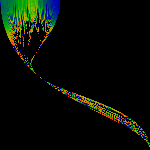Magic Third As above, except only the range 1/2 < x < 2/3 is shown (not to scale).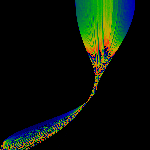The Brush A different 1/2 < x < 2/3 map.

Road to the Horizon If x = 1/(a1+1/(a2+1/(a3+1/(a4+ ...)))) then consider hg(x) = 1/(g(a1)+1/(g(a2)+1/(g(a3)+1/(g(a4)+ ...)))) In the road to the horizon, we use g(z)=1/z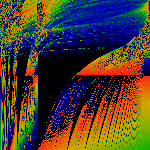Phat A 'trivial' reworking of the map: this shows x fz(x). Note since f1(x) = x, that the bottom row of pixels is just x2.Crystalline An attempt to create a symmetrized version.

These show the basic map on an extended range ... either wider (x runs zero to two) or taller (z runs zero to 4), or both. Note that we accidentally flipped some of these "upside down".

## Continued Crunch

These pictures depict the actual values that the function ranges over.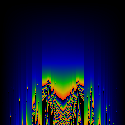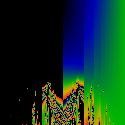The basic map, which is combined with itself to show the symmetric and the anti-symmetric components. The color at pixel (x,z) is simply the value of the fz(x) mod 1. Again, black=0.0, red=1.0, and a spectrum from blue to green in between. Note that z runs from +1 at the top, to -1 downwards. Thus, the top edge of the first picture is simple the straight line f1(x)=x.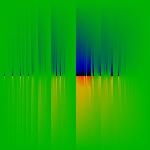As above, but this time, z=eit with t running from 0 to 2pi bottom to top. The first one shows the modulus, the second one the phase. Note how the phase picture confirms the existence of the poles at z=-1. The phases are colored with red=+pi, black=-pi, and green=0.

## Glossary

Farey Transform
The term 'Farey Transform' is idiosyncratic on my part, I don't believe anyone else calls it that (I don't know what they might call it). I call it that because of its deep relationship to the Farey Tree, as pointed out by John Conway. The web page Gap Theory provides a theoretical treatment in such a way that it becomes clear in what ways these functions are well-defined, analytic, and etc.

Hausdorff Measure
When we say 'Hausdorff Measure' above, what we really mean is this: Take the real number line between 0 and 1, and divide it into N equal bins. We start with each bin being empty (zero). Next, generate a sequence of random numbers 'x' between 0 and 1. Apply a transform (f(x) mod 1) to each x. Then, if f(x) falls in the i'th bin, increment the value of the i'th bin by one. When properly normalized, the resulting 'density' on the real-number line converges to a stable limit as N is increased, and as the number of samples is increased. We loosely call this value of the density the 'Hausdorff measure', in that it describes a practical way of taking a well-defined limit.

Most of these images were generated during January and February of 1994, in Austin, Texas. The work was inspired by a Christmas reading of the "Contorted Fractions" chapter of John Conway's "On Numbers and Games". The importance of Farey Trees to fractal phenomena was previously brought to my attention by Paul Cvitanovi\'c during some lectures in Paris in 1985.

Linas Vepstas February 1994

## References

• Stern-Brocot Tree
• Picture of a Farey Tree
• The Rational Mean as presented by Domingo Gómez Morín is a particularly fascinating, forceful, and instructive generalization of the ideas behind Farey addition. It in turn leads to the concept of a Rational Process, which defines a set of sequences of ratios that can be made to converge to ... well, I'm not sure ... not only the golden mean, but also to any simple root ... seems like these ideas might unify a variety of different concepts...
• The Minkowski Question Mark provides another important bridge between Farey Trees and Fractals.
• Designing with Farey Fractions reviews how to weave Farey Fractions into cloth! C'est fantastique!
The quickie bibliography below was scammed from http://www.math.uwn.edu/Farey.html

1. J.C. Lagarias and C. Tresser, A walk along the branches of the extended Farey tree, IBM Jour. of Res. and Dev., v. 39, 1995.
2. J.C. Lagarias, Number theory and dynamical systems, Proceedings of Symposia in Applied Mathematics 46, 35-72, 1992.
3. R. Siegel, C. Tresser, and G. Zettler, A decoding problem in dynamics and in number theory, Chaos 2, 473-493, 1992.
4. G.H. Hardy and E.M. Wright, An introduction to the theory of numbers, fifth edition, Clarendon, Oxford, England, 1979.
5. J. Farey, On a curious property of vulgar fractions, Philos. Mag. & Journal, London 47, 385-386, 1816.
6. Anonymous author, On vulgar fractions, Philos. Mag. & Journal, London 48, 204, 1816.
7. J. Farey, Propriété curieuse des Fractions Ordinaires, Bull. Sc. Soc. Philomatique 3, No. 3, 112, 1816.
8. J. Franel, Les Suites de Farey et le Problémes des Nombres Premiers, Gottinger Nachrichten, pp. 198-201, 1924.
9. P. Cvitanovi\'c, Farey Organization of the fractal hall effect, Phys. Scripta T9, 202, 1985.
10. P. Cvitanovi\'c, B. Shraiman, and B. Söderberg, Scaling laws for mode locking in circle maps, Phys. Scripta 32, 263-270, 1985.